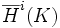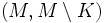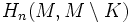# Alexander duality theorem

Let$M$ be an orientable manifold and$K$ a compact subset of$M$. Denote by$\overline{H}^i(K)$ the direct limit of cohomology groups for all open sets containing$K$. Suppose$(M,M \setminus K)$ is$R$-orientable. Choose a generator for$H_n(M, M \setminus K)$ (this group is a free module of rank one over the coefficient ring). Then cap product with this generator yields a map:$\overline{H}^i(K; R) \to H_{n-i}(M, M \setminus K ; R)$
Note that the specific isomorphism depends on the choice of orientation on the pair$(M, M \setminus K)$.